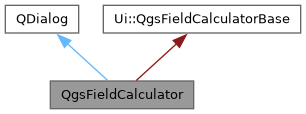QGIS API Documentation 3.35.0-Master (728e5967df5)
Searching...
No Matches
QgsFieldCalculator Class Reference

A dialog class that provides calculation of new fields using existing fields, values and a set of operators. More...

`#include <qgsfieldcalculator.h>`

Inheritance diagram for QgsFieldCalculator:[legend]

## Public Slots

void accept () override

## Public Member Functions

QgsFieldCalculator (QgsVectorLayer *vl, QWidget *parent=nullptr)

int changedAttributeId () const
Returns the field index of the field for which new attribute values were calculated.

## Friends

class TestQgsFieldCalculator

## Detailed Description

A dialog class that provides calculation of new fields using existing fields, values and a set of operators.

Sample usage of the QgsFieldCalculator class:

uri = "point?crs=epsg:4326&field=id:integer"
layer = QgsVectorLayer(uri, "Scratch point layer", "memory")
layer.startEditing()
dialog = QgsFieldCalculator(layer)
dialog.exec_()
A dialog class that provides calculation of new fields using existing fields, values and a set of ope...
Represents a vector layer which manages a vector based data sets.

Definition at line 42 of file qgsfieldcalculator.h.

## ◆ QgsFieldCalculator()

 QgsFieldCalculator::QgsFieldCalculator ( QgsVectorLayer * vl, QWidget * parent = `nullptr` )

Definition at line 46 of file qgsfieldcalculator.cpp.

## ◆ accept

 void QgsFieldCalculator::accept ( )
overrideslot

Definition at line 167 of file qgsfieldcalculator.cpp.

## ◆ changedAttributeId()

 int QgsFieldCalculator::changedAttributeId ( ) const
inline

Returns the field index of the field for which new attribute values were calculated.

Returns
The field index if attribute values were calculated or -1, e.g. in case of geometry changes.

Definition at line 53 of file qgsfieldcalculator.h.

## ◆ TestQgsFieldCalculator

 friend class TestQgsFieldCalculator
friend

Definition at line 89 of file qgsfieldcalculator.h.

The documentation for this class was generated from the following files: